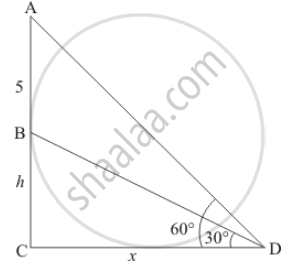Share

# A Vertical Tower Stands on a Horizontal Plane and is Surmounted by a Vertical Flag-staff of Height 5 Meters. at a Point on the Plane, the Angles of Elevation of the Bottom and the Top of the Flag-staff Are Respectively 300 and 600. Find the Height of the Tower. - CBSE Class 10 - Mathematics

#### Question

A vertical tower stands on a horizontal plane and is surmounted by a vertical flag-staff of height 5 meters. At a point on the plane, the angles of elevation of the bottom and the top of the flag-staff are respectively 300 and 600. Find the height of the tower.

#### Solution

Let BC be the tower of height, hm and AB be the Flagstaff with distance 5m. Then the angle of elevation from the top and bottom of Flagstaff are 60° and 30° respectively.

Let CD= x and ∠ADC = 60^@ ∠BDC = 30°

Here we have to find height of tower.So we use trigonometric ratios.

In a triangle BCD

=> tan D = (BC)/(CD)

=> tan 30^@ = h/x

=> 1/sqrt3 = h/x

=> x =  sqrt3h

Again in a triangle ACD

=> tan D = (AB + BC)/(CD)

=> tan 60^@ = (h + 5)/x

=> sqrt3 = (h + 5)/x

=> sqrt3x = h + 5

=> sqrt3 xx hsqrt3 = h + 5

=> 3h = h + 5

=> 2h = 5

=> h = 2.5

Hence the height of tree is 2.5 m

Is there an error in this question or solution?

#### Video TutorialsVIEW ALL 

Solution A Vertical Tower Stands on a Horizontal Plane and is Surmounted by a Vertical Flag-staff of Height 5 Meters. at a Point on the Plane, the Angles of Elevation of the Bottom and the Top of the Flag-staff Are Respectively 300 and 600. Find the Height of the Tower. Concept: Heights and Distances.
S